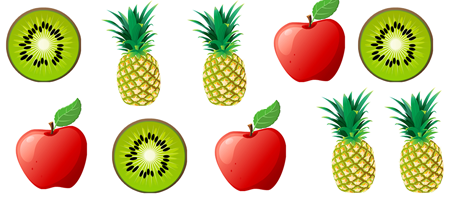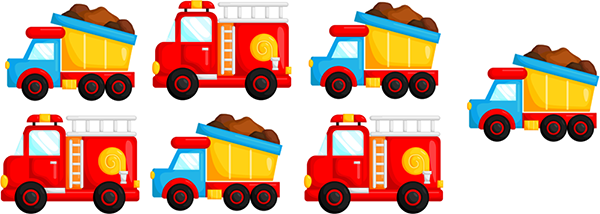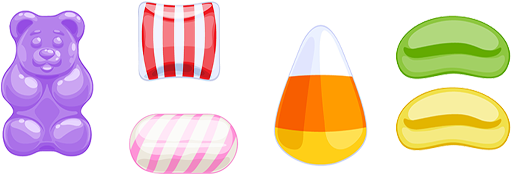# Counting Objects That are Different

Description: Teach students that objects of different types can be counted together.

Tags: Kindergarten, Counting, Counting to 10, Preschool, CCSS.MATH.CONTENT.K.CC.B.4.B, Kindergarten Math Problems

Question 1 (Short Answer, 10 points)

How many fruits can you count below?Question 2 (Multiple Answer, 10 points)

How many trucks are below?Question 3 (Multiple Answer, 10 points)

How many signs are there below?Question 4 (Multiple Choice, 10 points)

How many candies are below?Question 5 (Short Answer, 10 points)

How many balloons are below?